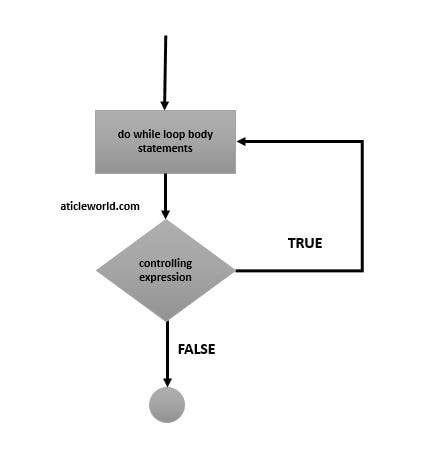# do while loop in C

do while loop (Iteration statement) execute a statement or block of statements until the given condition is true.

Syntax:

do
{
//do while body
}while ( expression ) ;

### Flow-chart of the do while loop in C

In the do while, evaluation of the controlling expression takes place after each execution of the do while body. So, that is the reason body of the do while is always executed at least once.### How does the do while loop work?

Step 1.  The body of the do while is executed.

Step 2. The do while evaluates the controlling expression. If the controlling expression is false, then control jumps to the next statement in the program (exit from the loop).

Step 3. If the controlling expression is true or any non zero value, then the process is repeated from step 1.

For example,

In the below example, I am printing and calculating the table of 10.

Output:Similar the while loop, we can also terminate do while using the break, goto or return keyword. We can also terminate an iteration without exiting the do while loop using the continue keyword.

for example,

Output:

Value of loop = 0
Value of loop = 1

### When should we use do while loop?

We use the do while loop where we want to execute the body of the loop at least once. Suppose a scenario where the program wants only positive value from the user and wait until the user does not enter the positive value. In that scenario do while is the best choice for the programmer.

See the below sample code,

do
{
//Get value from the user
value = getInputValue();
}while(value < 0)